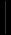## The Mass of Pluto

 Pluto is tiny - but what do we know of its mass. Pluto is a very tiny planet in space. Even if you observe the sky with the largest telescopes, it appears as a tiny dot. Furthermore, there is so little information about Pluto, so it’s hard to determine its mass by just looking and studying it. Therefore, the only method that can be used to measure its mass is its brightness. Astronomers have been working hard to find out the mass by knowing how bright it is. Through many studies, the astronomers have discovered that there are large quantities of methane ice on its surface. Therefore, Pluto had to be very bright. Since it was very bright, the astronomers guessed that it was larger than Mercury or the Earth’s Moon. However, this prediction was proven wrong by James Christy. In 1978, James discovered Pluto’s moon, Charon. It is easy to find out the precise mass once the astronomers find the planet’s moon. They can use Newton’s formulation of Kepler’s Law to calculate the mass once there is a system where two objects, Pluto and Charon, are orbiting one another. After the calculation, the mass came out to be a number that was much smaller than astronomer’s prediction. Newton’s formulation gave 1.31 x 1022 kg, which is only 0.24%, the mass of Earth. Later on, with more precise observations, the astronomers found out that the diameter of Pluto is 2,390 km across.

Featured:
Daily Moon Horoscope